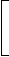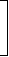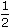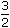# Aptitude - Banker's Discount

Exercise : Banker's Discount - General Questions
1.
The banker's discount on a bill due 4 months hence at 15% is Rs. 420. The true discount is:
Rs. 400
Rs. 360
Rs. 480
Rs. 320
Explanation:
T.D.
 = B.D. x 100 100 + (R x T)
= Rs.420 x 100100 +15 x 13
 = Rs.420 x 100105
= Rs. 400.

2.
The banker's discount on Rs. 1600 at 15% per annum is the same as true discount on Rs. 1680 for the same time and at the same rate. The time is:
3 months
4 months
6 months
8 months
Explanation:

S.I. on Rs. 1600 = T.D. on Rs. 1680.Rs. 1600 is the P.W. of Rs. 1680, i.e., Rs. 80 is on Rs. 1600 at 15%.Time =100 x 80year = 1 year = 4 months. 1600 x 15 3

3.
The banker's gain of a certain sum due 2 years hence at 10% per annum is Rs. 24. The present worth is:
Rs. 480
Rs. 520
Rs. 600
Rs. 960
Explanation:

 T.D. =B.G. x 100= Rs.24 x 100= Rs. 120. Rate x Time 10 x 2P.W. = 100 x T.D. = Rs.100 x 120= Rs. 600. Rate x Time 10 x 2

4.
The banker's discount on a sum of money for 1years is Rs. 558 and the true discount on the same sum for 2 years is Rs. 600. The rate percent is:
10%
13%
12%
15%
Explanation:

B.D. foryears = Rs. 558.
B.D. for 2 years
 = Rs.558 x 2 x 23
= Rs. 744

T.D. for 2 years = Rs. 600.Sum = B.D. x T.D. = Rs.744 x 600= Rs. 3100. B.D. - T.D 144

Thus, Rs. 744 is S.I. on Rs. 3100 for 2 years.Rate =100 x 744% = 12% 3100 x 2

5.
The banker's gain on a sum due 3 years hence at 12% per annum is Rs. 270. The banker's discount is:
Rs. 960
Rs. 840
Rs. 1020
Rs. 760
 T.D. =B.G. x 100= Rs.270 x 100= Rs. 750. R x T 12 x 3B.D. = Rs.(750 + 270) = Rs. 1020.